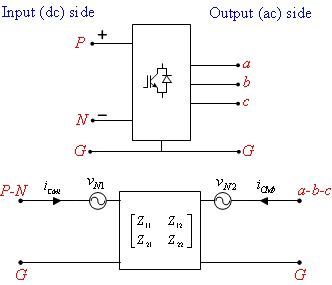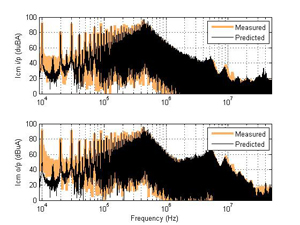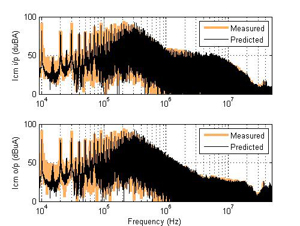RESEARCH

# public/showcase/2013_T4.4.phpFig. 1. CM noise models for a motor-drive system.
Terminal electromagnetic interference (EMI) models of power converters have been shown to successfully predict conducted emissions of up to at least 30 MHz. For a fixed load, these models can accurately predict the input side of EMI converters for changes in the source impedance. In other words, these models cannot predict the input side EMI for changes in the load side parameters. Such terminated EMI models have limited utility as they cannot be used for converters that have a strong coupling between the EMI input and output side. Moreover, in aerospace applications, the conducted EMI standards (DO-160) dictate limits on the noise currents in all power lines and cable bundles, thus compelling development of models that can predict the EMI on both sides of the power converters. The proposed un terminated EMI terminal model in this paper directly predicts the input and output side common mode (CM) noise currents in a motor drive system for changes in both source and load side impedances. Such models can be very useful in understanding how EMI filters and harnesses affect the overall EMI in a motor drive system.

The model is based on the Thevenin equivalent of a three-terminal network and is shown in Fig. 1. The model's impedance matrix is approximated directly using measurements under power-off conditions and then optimized using noise measurements done while the drive is in operation. The noise sources are approximated using in-circuit measurements. The prediction accuracy of the input and output side CM currents when the input LISN impedance is changed from 50ω to 1kω is shown in Fig. 2. Fig. 3 shows the prediction accuracy with a 50ω LISN at the input side and a DM filter at the output. It can be clearly seen that the model can predict both input and output side CM currents up to 30 MHz.Fig. 2. CM noise prediction for a 1kω LISN.Fig. 3. CM noise prediction for an output side DM filter.
INDUSTRY PARTNERS